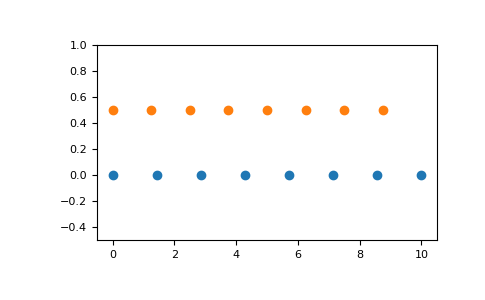# numpy.linspace¶

`numpy.``linspace`(start, stop, num=50, endpoint=True, retstep=False, dtype=None, axis=0)[source]

Return evenly spaced numbers over a specified interval.

Returns num evenly spaced samples, calculated over the interval [start, stop].

The endpoint of the interval can optionally be excluded.

Changed in version 1.16.0: Non-scalar start and stop are now supported.

Parameters
startarray_like

The starting value of the sequence.

stoparray_like

The end value of the sequence, unless endpoint is set to False. In that case, the sequence consists of all but the last of `num + 1` evenly spaced samples, so that stop is excluded. Note that the step size changes when endpoint is False.

numint, optional

Number of samples to generate. Default is 50. Must be non-negative.

endpointbool, optional

If True, stop is the last sample. Otherwise, it is not included. Default is True.

retstepbool, optional

If True, return (samples, step), where step is the spacing between samples.

dtypedtype, optional

The type of the output array. If `dtype` is not given, infer the data type from the other input arguments.

New in version 1.9.0.

axisint, optional

The axis in the result to store the samples. Relevant only if start or stop are array-like. By default (0), the samples will be along a new axis inserted at the beginning. Use -1 to get an axis at the end.

New in version 1.16.0.

Returns
samplesndarray

There are num equally spaced samples in the closed interval `[start, stop]` or the half-open interval `[start, stop)` (depending on whether endpoint is True or False).

stepfloat, optional

Only returned if retstep is True

Size of spacing between samples.

`arange`

Similar to `linspace`, but uses a step size (instead of the number of samples).

`geomspace`

Similar to `linspace`, but with numbers spaced evenly on a log scale (a geometric progression).

`logspace`

Similar to `geomspace`, but with the end points specified as logarithms.

Examples

```>>> np.linspace(2.0, 3.0, num=5)
array([2.  , 2.25, 2.5 , 2.75, 3.  ])
>>> np.linspace(2.0, 3.0, num=5, endpoint=False)
array([2. ,  2.2,  2.4,  2.6,  2.8])
>>> np.linspace(2.0, 3.0, num=5, retstep=True)
(array([2.  ,  2.25,  2.5 ,  2.75,  3.  ]), 0.25)
```

Graphical illustration:

```>>> import matplotlib.pyplot as plt
>>> N = 8
>>> y = np.zeros(N)
>>> x1 = np.linspace(0, 10, N, endpoint=True)
>>> x2 = np.linspace(0, 10, N, endpoint=False)
>>> plt.plot(x1, y, 'o')
[<matplotlib.lines.Line2D object at 0x...>]
>>> plt.plot(x2, y + 0.5, 'o')
[<matplotlib.lines.Line2D object at 0x...>]
>>> plt.ylim([-0.5, 1])
(-0.5, 1)
>>> plt.show()
```numpy.arange

numpy.logspace